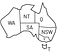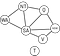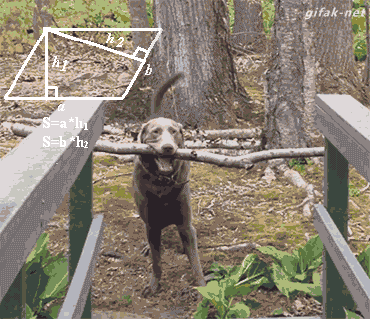# AI Fundamentals: Introduction to Constraints Satisfaction Problems (CSP)Photo by Ondrej Machart on Unsplash

Anyone who wants to seriously approach the study of Artificial Intelligence starts by studying the Constraints Satisfaction Problem. In this article, I will introduce you to CSPs: I will explain what they are, how they can be useful to you, and how to solve them, through a practical example derived directly from the kangaroo nation 🦘.

Nowadays, CSPs are used in many fields, such as biology (DNA sequencing), Constraint Databases, Diagnosis, Natural Language Recognition, and so on.

Constraint Satisfaction Problems (CSP) represents a class of problems where there are some restrictions between objects within that problem.

In a formal way, a CSP is composed of three components:
1. A set of variables (V = {V1…Vn})
2. A domain for each variables (D = {D1 … Dn})
3. A set of constraints ( C )

Like every problem, also CSP can have solutions; but we need to define the “notion of solution”. So, let’s start from states:

A state in a CSP is an assignment of values to some or all of the variables; we define as partial assignment in the first case (where not every variable has an assignment) and complete assignment in the other case. We also define a consistent assignment as one assignment that satisfies all the constraints.

A solution to a CSP (or the goal state) is a complete and consistent assignment.If you didn’t know before, there are these 7 regions in Australia

Finally, we can see an example. This is the famous “Australia map coloring problem” and it can be described as following:

You have to color each region of Australia using three colors {red, blue green} so that no neighboring territory has the same color.

Ok, it can be even too simple at first glance but let’s define the CSP:
1. V = {WA, NT, Q, NSW, V, SA, T}
2. D = {green, red, blue}, in this problem all variables have the same domain
3.
C = {“adjacent regions can’t have the same color”}The Constraint Graph

The first thing we can do to better understand this problem is to define the constraint graph, which is useful to view the relations among variables.
The constraint graph is an undirected graph where we put a node for each variable (from the original CSP) and an edge between two nodes if there is a constraint among the variables represented by the nodes.

Ok now, we can think about finding some solutions for our problem.We. Now.

Have you ever heard of a brute force hacking attack? Here, it’s the same principle. This strategy provides to generate a complete assignment to each variable, check if that assignment is a solution, checking if each constraint is satisfied. If so, this strategy has found a solution, if not, start again. This is not the best strategy because there is a lot of useless work. Let’s think of a smarter solution instead.

This strategy involves checking the constraint after each assignment on a single variable — and not after each variable is assigned like the Generate-and-Test strategy.
So, if some constraints are violated, this strategy backtracks to previous choices (undoing the assignment) and tries another assignment.

In the Australian problem we could have this behavior:
The strategy starts with the empty assignment, picks WA as first choice (it is not important where to start). WA has three possibilities (red, blue, or green).
The strategy chooses one assignment and it performs the constraint check. If there is a violation of a constraint, it reverts (or, backtracks) the assignment and tries with another assignment. Pretty simple!When the algorithm found no solution:

There’s so much more to say about CSPs, if you enjoyed this article and are interested in more articles of the genre I ask you to hit the clap button to let me know.
And if you like, you can give me your feedback on Telegram!

🇪🇺 European Android Developer

## More from Gennaro Daniele Acciaro

🇪🇺 European Android Developer